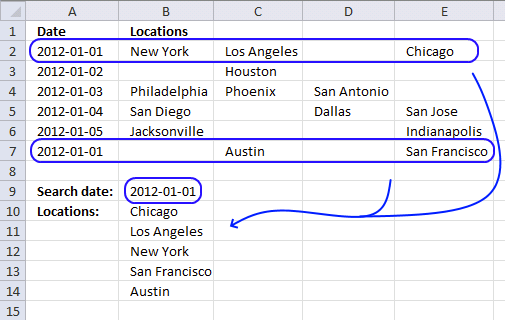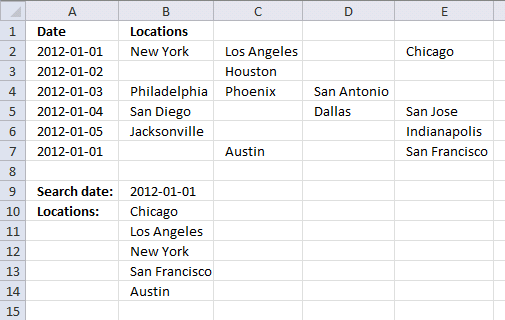Author: Oscar Cronquist Article last updated on November 08, 2019This article demonstrates a formula that lets you extract non-empty values across columns based on a condition. The image above shows the condition in cell B9 and the formula in cell range B10:B14.

The data set is in cell range A2:E7 and the lookup column is column A. The formula returns values from multiple rows if the corresponding value in the lookup column match, one value in each cell.

I geted the file lookup-vba3. I think I can use this to help me populate a calendar.I substituted dates for Pen, Paper, and Eraser. I then had locations substituted for \$ values. Where I have a date of say, 11/27/12, I have 10 locations delivering that day.

Using the template as shown in the screenshot under "Return multiple values horizontally or vertically (VBA)".

I cannot expand past column "C" to return multiple values. I think it is in the array code but I cannot figure out how to return values past column C.

If you can help, greatly appreciated!

Thanks,
Jim

Array formula in cell B10:

=IFERROR(INDEX(\$B\$2:\$E\$7, SMALL(IF((\$A\$2:\$A\$7=\$B\$9)*(\$B\$2:\$E\$7<>""), MATCH(ROW(\$B\$2:\$E\$7), ROW(\$B\$2:\$E\$7)), ""), ROW(A1)), 1/(SMALL(IF((\$A\$2:\$A\$7=\$B\$9)*(\$B\$2:\$E\$7<>""), MATCH(ROW(\$B\$2:\$E\$7), ROW(\$B\$2:\$E\$7))+1/MATCH(COLUMN(\$B\$2:\$E\$7), COLUMN(\$B\$2:\$E\$7)), ""), ROW(A1))-SMALL(IF((\$A\$2:\$A\$7=\$B\$9)*(\$B\$2:\$E\$7<>""), MATCH(ROW(\$B\$2:\$E\$7), ROW(\$B\$2:\$E\$7)), ""), ROW(A1)))), "")

How to create an array formula

1. Select cell B10.
2. Press with left mouse button on in formula bar.
3. Paste above array formula.
4. Press and hold CTL + SHIFT simultaneously.
5. Press Enter.

How to copy formula

1. Select cell B10.
2. Copy cell (Ctrl + c).
3. Select cell range B11:B15.
4. Paste (Ctrl + v).

The following array formula concatenates the returned values, the TEXTJOIN function is able to make the formula much smaller.

Array formula in cell B10:

=TEXTJOIN(" ,",TRUE,IF((A2:A7=B9)*(B2:E7<>""),B2:E7,""))

### Explaining array formula in cell B10The INDEX function returns a value or a reference of the cell at the intersection of a particular column and row, in a given range.

INDEX(\$B\$2:\$E\$7, row_num, column_num)

The first following three steps calculate the row_nums and the remaining steps calculate column_nums.

#### Step 1 - Find matching dates and non blanks

The equal sign is a logical operator. it lets you compare the value in cell B9 with cell range A2:A7, the logical expression returns TRUE if equal and FALSE if not.

\$A\$2:\$A\$7=\$B\$9

becomes

{40909; 40910; 40911; 40912; 40913; 40909}=40909

becomes

{TRUE; FALSE; FALSE; FALSE; FALSE; TRUE}

The less than and greater than characters are also logical operators, they check if values in cell range B2:E7 are not blank.

\$B\$2:\$E\$7<>""

becomes

{"New York","Los Angeles",0,"Chicago"; 0,"Houston",0,0; "Philadelphia","Phoenix","San Antonio",0; "San Diego",0,"Dallas","San Jose"; "Jacksonville",0,0,"Indianapolis"; 0,"Austin",0,"San Francisco"}<>""

becomes

{TRUE, TRUE, TRUE, TRUE;TRUE, TRUE, TRUE, TRUE;TRUE, TRUE, TRUE, TRUE;TRUE, TRUE, TRUE, TRUE;TRUE, TRUE, TRUE, TRUE;TRUE, TRUE, TRUE, TRUE}

The parentheses allow you to manipulate the order of calculation which is really important in this step. The asterisk is a character that multiplies the two arrays, TRUE*TRUE = TRUE (1), TRUE*FALSE = FALSE (0) and FALSE * FALSE = FALSE (0). This means that AND logic is applied to the two arrays.

You can multiply arrays with different sizes as long as you follow certain rules, in this case, I am multiplying an array that has the same number of rows as the other array.

(\$A\$2:\$A\$7=\$B\$9)*(\$B\$2:\$E\$7<>"")

returns

{1, 1, 0, 1;0, 0, 0, 0;0, 0, 0, 0;0, 0, 0, 0;0, 0, 0, 0;0, 1, 0, 1}.

1 is the same as TRUE and 0 (zero) is FALSE. Excel converts the boolean values to their numerical equivalents when you perform arithmetic calculations between two or more arrays.

#### Step 2 - Return corresponding row numbers

IF((\$A\$2:\$A\$7=\$B\$9)*(\$B\$2:\$E\$7<>""), MATCH(ROW(\$B\$2:\$E\$7), ROW(\$B\$2:\$E\$7)), "")

becomes

IF({1, 1, 0, 1;0, 0, 0, 0;0, 0, 0, 0;0, 0, 0, 0;0, 0, 0, 0;0, 1, 0, 1}, {1;2;3;4;5;6}, "")

and returns

{1, 1, "", 1;"", "", "", "";"", "", "", "";"", "", "", "";"", "", "", "";"", 6, "", 6}

Recommended articles

How to use the IF function
Checks if a logical expression is met. Returns a specific value if TRUE and another specific value if FALSE.

#### Step 3 - Return the k-th smallest value

SMALL(array, k)

SMALL(IF((\$A\$2:\$A\$7=\$B\$9)*(\$B\$2:\$E\$7<>""), MATCH(ROW(\$B\$2:\$E\$7), ROW(\$B\$2:\$E\$7)), ""), ROW(A1))

becomes

SMALL({1, 1, "", 1;"", "", "", "";"", "", "", "";"", "", "", "";"", "", "", "";"", 6, "", 6}, 1)

and returns 1.

Recommended articles

How to use the SMALL function
The SMALL function lets you extract a number in a cell range based on how small it is compared to the other numbers in the group.

#### Step 1 - Find matching dates and non blanks

(\$A\$2:\$A\$7=\$B\$9)*(\$B\$2:\$E\$7<>"")

becomes

({40909; 40910; 40911; 40912; 40913; 40909}=40909)*({"New York","Los Angeles",0,"Chicago"; 0,"Houston",0,0; "Philadelphia","Phoenix","San Antonio",0; "San Diego",0,"Dallas","San Jose"; "Jacksonville",0,0,"Indianapolis"; 0,"Austin",0,"San Francisco"}<>"")

and returns

{1, 1, 0, 1;0, 0, 0, 0;0, 0, 0, 0;0, 0, 0, 0;0, 0, 0, 0;0, 1, 0, 1}

#### Step 2 - Calculate both row numbers and column numbers

IF((\$A\$2:\$A\$7=\$B\$9)*(\$B\$2:\$E\$7<>""), MATCH(ROW(\$B\$2:\$E\$7), ROW(\$B\$2:\$E\$7))+1/MATCH(COLUMN(\$B\$2:\$E\$7), COLUMN(\$B\$2:\$E\$7)), "")

becomes

IF({1, 1, 0, 1;0, 0, 0, 0;0, 0, 0, 0;0, 0, 0, 0;0, 0, 0, 0;0, 1, 0, 1}, {1;2;3;4;5;6}+{1,0.5,0.333333333333333,0.25}, "")

and returns

{2,1.5,"",1.25;"","","","";"","","","";"","","","";"","","","";"",6.5,"",6.25}

#### Step 3 - Return the k-th smallest value

SMALL(array, k)

SMALL(IF((\$A\$2:\$A\$7=\$B\$9)*(\$B\$2:\$E\$7<>""), MATCH(ROW(\$B\$2:\$E\$7), ROW(\$B\$2:\$E\$7))+1/MATCH(COLUMN(\$B\$2:\$E\$7), COLUMN(\$B\$2:\$E\$7)), ""), ROW(A1))

becomes

SMALL({2,1.5,"",1.25;"","","","";"","","","";"","","","";"","","","";"",6.5,"",6.25}, 1)

and returns 1.25.

#### Step 4 - Subtract row numbers

SMALL(IF((\$A\$2:\$A\$7=\$B\$9)*(\$B\$2:\$E\$7<>""), MATCH(ROW(\$B\$2:\$E\$7), ROW(\$B\$2:\$E\$7))+1/MATCH(COLUMN(\$B\$2:\$E\$7), COLUMN(\$B\$2:\$E\$7)), ""), ROW(A1))-SMALL(IF((\$A\$2:\$A\$7=\$B\$9)*(\$B\$2:\$E\$7<>""), MATCH(ROW(\$B\$2:\$E\$7), ROW(\$B\$2:\$E\$7)), ""), ROW(A1))

becomes

SMALL({2,1.5,"",1.25;"","","","";"","","","";"","","","";"","","","";"",6.5,"",6.25}, 1)-SMALL({1, 1, "", 1;"", "", "", "";"", "", "", "";"", "", "", "";"", "", "", "";"", 6, "", 6}, ROW(A1))

becomes

1.25-1

and returns 0.25

#### Step 5 - Calculate column number

1/(SMALL(IF((\$A\$2:\$A\$7=\$B\$9)*(\$B\$2:\$E\$7<>""), MATCH(ROW(\$B\$2:\$E\$7), ROW(\$B\$2:\$E\$7))+1/MATCH(COLUMN(\$B\$2:\$E\$7), COLUMN(\$B\$2:\$E\$7)), ""), ROW(A1))-SMALL(IF((\$A\$2:\$A\$7=\$B\$9)*(\$B\$2:\$E\$7<>""), MATCH(ROW(\$B\$2:\$E\$7), ROW(\$B\$2:\$E\$7)), ""), ROW(A1)))

becomes

1/0.25 and returns column number 4.

#### Final calculation in cell B10

The INDEX function uses the row and column number to determine which value to return.

IFERROR(INDEX(\$B\$2:\$E\$7, SMALL(IF((\$A\$2:\$A\$7=\$B\$9)*(\$B\$2:\$E\$7<>""), MATCH(ROW(\$B\$2:\$E\$7), ROW(\$B\$2:\$E\$7)), ""), ROW(A1)), 1/(SMALL(IF((\$A\$2:\$A\$7=\$B\$9)*(\$B\$2:\$E\$7<>""), MATCH(ROW(\$B\$2:\$E\$7), ROW(\$B\$2:\$E\$7))+1/MATCH(COLUMN(\$B\$2:\$E\$7), COLUMN(\$B\$2:\$E\$7)), ""), ROW(A1))-SMALL(IF((\$A\$2:\$A\$7=\$B\$9)*(\$B\$2:\$E\$7<>""), MATCH(ROW(\$B\$2:\$E\$7), ROW(\$B\$2:\$E\$7)), ""), ROW(A1)))), "")

becomes

IFERROR(INDEX(\$B\$2:\$E\$7, 1, 4), "")

becomes

IFERROR("Chicago", "")

and returns "Chicago" in cell B10.

If the INDEX function returns an error value the IFERROR function catches the error and returns a blank "".

Note, use the IFERROR with caution. It handles all errors which may make it very difficult to spot errors in your formulas.

### Get the Excel fileLookup-and-return-multiple-values-from-a-range-excluding-blanks.xlsx